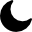EN# MATH

MATH
MATH Price
\$0.105
0.22%
Price range last 24 hours
\$0.103
Low
\$0.107
High
Tags
Rank #699
Cosmos Ecosystem
Polygon Ecosystem
Software
BNB Chain Ecosystem
Ethereum Ecosystem
SHA-256
Website
Explorers
Community
\$16.909,106
-0.28%
Market Cap
\$323.048
\$0
Circulating Supply
161.287,670 / 200.000,000
Total / Max Supply
MATH to USD Price Chart

## MATH Price Today

Price MATH to USD now is \$0.105. Trading volume by 24-hours \$323.048. MATH, which_ranked #699 price is up 0.22% in the past 24-hours. MATH has circulating supply of \$0 and have \$16.909,106 capitalization. In additional, total supply is \$161.287,670.

## Highest price for MATH

The all-time high price of MATH is \$3.15, the record was set on the 0.

## Lowest price for MATH

MATH all-time low price is \$0.058, the record was set on 0.

## How much has the price of MATH changed lately?

7 days ago the price was \$0 , this changed the MATH price by -4.92595%

14 days ago the price was \$0 , this changed the MATH price by -10.27548%

30 days ago the price was \$0 , this changed the MATH price by 0.19929%

60 days ago the price was \$0 , this changed the MATH price by 13.28939%

1 days ago the price was \$0 , this changed the MATH price by -84.31886%

## What is MATH

MATH is a one stop crypto solution platform which contains MathWallet, MATH VPOS Pool, MathDEX, MATH dApp Store, MATH Staking, MATH Pay, MATH Chain, etc.